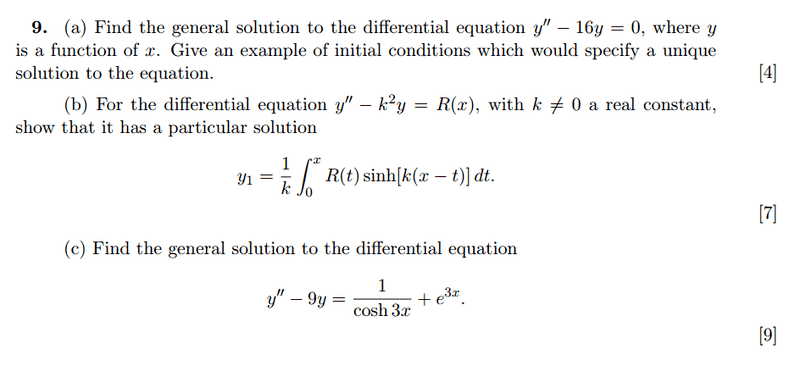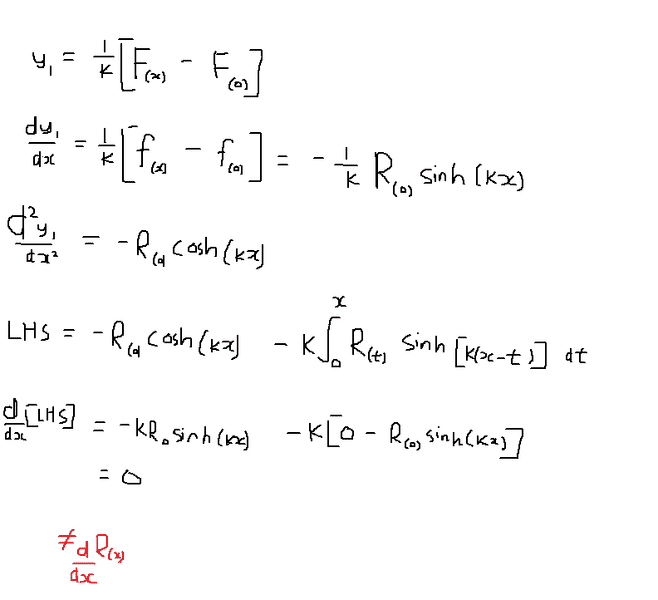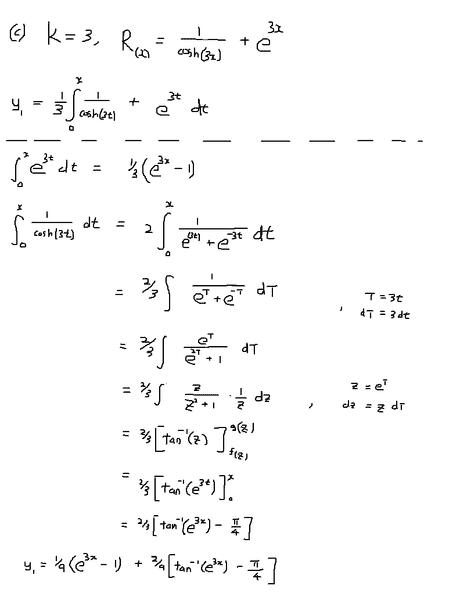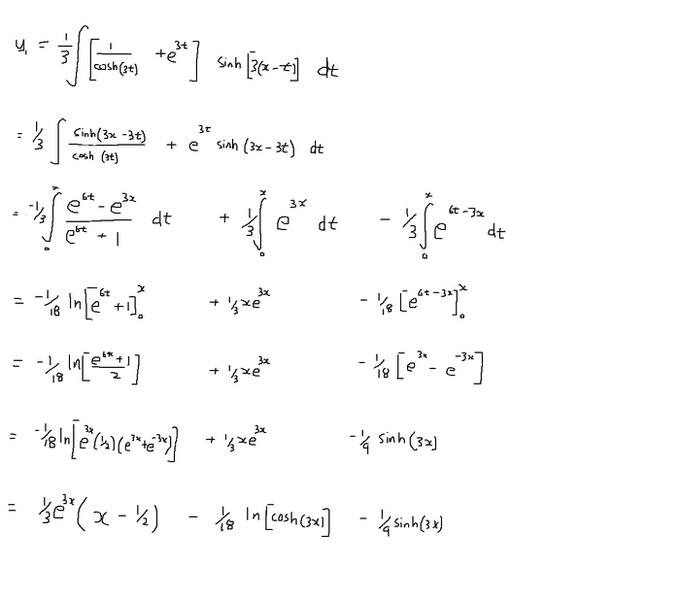# Solving this second order linear differential equation

## Homework Statement

Find the general solution to the differential equation y'' -16y = 0, where y is a function of x. Give initial conditions that would give a unique solution to the eqution.
For the differential equation y'' - k2y = R(x), with k ≠ 0 a real constant, show that it has a particular solution:

y1 = (1/k)∫R sinh[k(-t)] dt## The Attempt at a Solution

No problems with part (a) and finding homogeneous solution.

I tried to avoid expanding the integral using integration by parts by differentiating both LHS and RHS w.r.t. x. But I'm missing a dR/dx term...Is there anything wrong with my integration for part (c) below?Last edited:

Your derivative of $y_1$ is incorrect. Your integrand is a function of x (and t), and your limits of integration are functions of x. See the "differentiation under the integral sign" formula: http://en.wikipedia.org/wiki/Differentiation_under_the_integral_sign

For example, I find that $$\frac{dy_1}{dx}=\int_0^x R(t)\cosh(k(x-t))dt.$$

Your derivative of $y_1$ is incorrect. Your integrand is a function of x (and t), and your limits of integration are functions of x. See the "differentiation under the integral sign" formula: http://en.wikipedia.org/wiki/Differentiation_under_the_integral_sign

For example, I find that $$\frac{dy_1}{dx}=\int_0^x R(t)\cosh(k(x-t))dt.$$

don't you have to differentiate R too? since R is essentially a function of x just disguised by changing all the 'x' in the function to 't'

And, when i differentiate twice, it gives back the same integrand, and when subbed into LHS, it gives 0, instead of R.

Dick
Homework Helper
don't you have to differentiate R too? since R is essentially a function of x just disguised by changing all the 'x' in the function to 't'

And, when i differentiate twice, it gives back the same integrand, and when subbed into LHS, it gives 0, instead of R.

No, you don't differentiate R, it's not a function of x. Show how you differentiated twice. You are missing a part.

No, you don't differentiate R, it's not a function of x. Show how you differentiated twice. You are missing a part.

Differentiate once, times k and change sinh into cosh.

Differentiate second time, times k and change cosh into sinh.

resulting expression is k * oringinal..

Dick
Homework Helper
Differentiate once, times k and change sinh into cosh.

Differentiate second time, times k and change cosh into sinh.

resulting expression is k * oringinal..

You not only have to differentiate with respect to the x in the integrand, you also have to deal with the x in the limits of integration. That's where the R(x) is going to come from. See http://en.wikipedia.org/wiki/Leibniz_integral_rule The part you are missing is also known as the Fundamental Theorem of Calculus.

Last edited:
•1 person
You not only have to differentiate with respect to the x in the integrand, you also have to deal with the x in the limits of integration. That's where the R(x) is going to come from. See http://en.wikipedia.org/wiki/Leibniz_integral_rule The part you are missing is also known as the Fundamental Theorem of Calculus.

Thanks! I've worked out the answer! Strange enough, this isn't even in our syllabus or wasn't even taught in lectures. Didn't come across it in Riley Hobson and Bence as well..

You not only have to differentiate with respect to the x in the integrand, you also have to deal with the x in the limits of integration. That's where the R(x) is going to come from. See http://en.wikipedia.org/wiki/Leibniz_integral_rule The part you are missing is also known as the Fundamental Theorem of Calculus.

Is my integration at the last part correct?

Dick
Homework Helper
Is my integration at the last part correct?

I rather doubt it. Where's the sinh(3(x-t)) part?

I rather doubt it. Where's the sinh(3(x-t)) part?

I've corrected my working, not sure if it's right. Especially from line  to line . I'm not sure if i can take the ln [] of the bottom, given that there's both x and t, which works only if the reverse process is a partial derivative, ∂/∂t.

Dick
Homework Helper
I've corrected my working, not sure if it's right. Especially from line  to line . I'm not sure if i can take the ln [] of the bottom, given that there's both x and t, which works only if the reverse process is a partial derivative, ∂/∂t.

I still don't the see the integral you actually want to do. You want to integrate
$$\int_0^x (\frac{1}{\cosh(3t)}+e^{3t})(\cosh(3(x-t)) dt$$
Don't you?

I still don't the see the integral you actually want to do. You want to integrate
$$\int_0^x (\frac{1}{\cosh(3t)}+e^{3t})(\cosh(3(x-t)) dt$$
Don't you?

Oops, I forgot to attach my working. Here it is:

From line  to line  i'm not sure if i can take the ln [] of the bottom, given that there's both x and t, which works only if the reverse process is a partial derivative, ∂/∂t.Dick